# Support vector machines

The classification methods we have discussed so far can do well for data analytics, with relatively few predictors, when clarity and transparency are needed. As the goal gets more complex, features get less transparent and less interpretable, the number of examples get large, and number of classes get large, these properties will sometimes take a backseat to performance.

A very type of classifier advances over LDA and QDA, and is called a ‘support vector machine’. These are examples of the ‘kernel’ methods. In general, they are suitable for complex problems with relatively large training sets, and they rose to popularity following the limitations of early neural network models.

The internal methods of these classifiers are beyond the scope of this class, although we will discuss some of aspects. At a practical level, we can think of these as advanced classifiers that in fact are rooted in statistical decision theory from which LDA methods arose. Just like QDA allows a simple (quadratic) polynomial to form the boundary between classes, we might consider a more complex polynomial. One of the things SVMs allow is to create a complex boundary between classes in a high-dimensional space. This goal is what gives the SVM its name: if you can identify a set of points (a vector) on the boundary between classes in a high-dimensional space (including polynomial functions and products of the given features), this set provides the support to model a boundary. SVMs attempt to find a space in which they can optimally separate these points.

Some of these methods become a challenge as complexity scales large. These include: * Optimization. The least-squares approach used in regression, and maximum likelihood methods used in the glm may not scale well as the number of predictors get large. SVMs typically attempt to map the problem into a problem referred to as a ‘quadratic programming’ (QP) problem, which uses numerical methods to optimize the multi-variate function.

• Complex Features. For some types of data (such as audio, imagery, and video), we might be interested in creating complex features and use simple methods to classify. For example, to classify written text, we might identify lines in certain angles and combinations of these lines. To identify speech, we might try to translate into phonemes and then into sound patterns, and finally to words. In contrast, SVMs use what is referred to as a kernel–a way of measuring the similarity between any two exemplars. When a well-understood kernel is used, this permits substantial more efficiency. Furthermore, the useful features may be complex combinations or transformations of the raw features, which a kernel may make easier to manage.

• Decision bounds. For simple classifications, decision rules based on simple combinations of features were sufficient. As the exemplar space gets complex, the bounds will become non-linear. Rather than choosing a simple bound based on maxmimizing cross-validation accuracy, a decision boundary and appropriate transformations are chosen that maximize the gap between groups (whil miniimizing mis-classification). These transformations can include, essentially, automatically examining interactions between features and using polynomial transforms as well, to permit separating classes with curved bounds.

SVMs were first described in the 1960s, but rose to prominance in the 1990s as tools for machine vision, when they began supplanting the neural networks developed in the 1980s. Their advantage over simpler methods are likely to be greatest when you have large databases with many features–such as images or sounds, that are hard to make sense of otherwise. However, they can still be used on the simpler classification problems we have examined.

There are several SVM tools available within R. One is the svm function within e1071; another is the svmlight function within klaR, which is an interface to the SVMlight library. svmlight requires installing that library, so we will use the e1071 library for examples.

Let’s look at the engineering data set first. We will run a classifier on the engineering data first. Because the predicted variable is numeric, we will make it a factor so the svm knows to predict the class.

library(e1071)
library(DAAG)
joint$eng <- as.factor(joint$eng)
s1 <- svm(y = joint$eng, x = joint[, -1], scale = T) summary(s1)  Call: svm.default(x = joint[, -1], y = joint$eng, scale = T)

Parameters:
SVM-Type:  C-classification
cost:  1

Number of Support Vectors:  73

( 37 36 )

Number of Classes:  2

Levels:
0 1
confusion(joint$eng, predict(s1, data = joint)) Overall accuracy = 0.868 Confusion matrix Predicted (cv) Actual 0 1 0 0.868 0.132 1 0.132 0.868 First, consider the arguments we gave. We specified that the variables should be rescaled–this makes sense here because the variables are on substantially different scales. Next, the svm used C-classification. This is the default for predicting factor values–if you give a numeric value to predict, it will implement a regression. Next, it describes that it uses a radial kernel, or more specifically a radial basis function (RBF) kernel. This is one of the most popular kernels–especially for data like image classification. It is usually better than the linear kernels as it permits interactions between features, and although it shares some aspects with the polynomial kernel but it is better behaved. Different types of data (networks, sparse text-document matrices, images, etc.) are usually best fit by one or another of the available kernels. Next, the model specifies its ‘support vectors’. This is in a sense a number of parameters used to make the decisions. Although simpler, it might be worthwhile to examine a linear or polynomial kernel. s1.lin <- svm(y = joint$eng, x = joint[, -1], kernel = "linear", scale = T)
s1.lin

Call:
svm.default(x = joint[, -1], y = joint$eng, scale = T, kernel = "linear") Parameters: SVM-Type: C-classification SVM-Kernel: linear cost: 1 Number of Support Vectors: 67 confusion(joint$eng, (predict(s1.lin, data = joint)))
Overall accuracy = 0.697

Confusion matrix
Predicted (cv)
Actual     0     1
0 0.684 0.316
1 0.289 0.711
s1.pol <- svm(y = joint$eng, x = joint[, -1], kernel = "polynomial", scale = T) s1.pol  Call: svm.default(x = joint[, -1], y = joint$eng, scale = T, kernel = "polynomial")

Parameters:
SVM-Type:  C-classification
SVM-Kernel:  polynomial
cost:  1
degree:  3
coef.0:  0

Number of Support Vectors:  74
confusion(joint$eng, (predict(s1.pol))) Overall accuracy = 0.789 Confusion matrix Predicted (cv) Actual 0 1 0 1.000 0.000 1 0.421 0.579 The linear SVM does not do as well, while the polynomial performs similarly to the RBF kernel, except that it gets 100% accuracy on the 0-0 cases, and does much more poorly on the 1-1 case. But these are as good or better than any of the other methods. The C-classification has two parameters that control the solution, the cost (C) of mis-classification and $$\gamma$$. It is typical to use cross-validation to help determine reasonable values for these. This svm library will do n-fold crossvalidation by specifying a cross function. Note that the default parameters for cost were 1.0, and for $$\gamma$$ were 0.1, which is something like 1/(# of predictors). Let’s start by comparing cross-validated predictions of linear and RBF: s1.lin <- svm(y = joint$eng, x = joint[, -1], kernel = "linear", scale = T, cross = 10)
print(s1.lin)

Call:
svm.default(x = joint[, -1], y = joint$eng, scale = T, kernel = "linear", cross = 10) Parameters: SVM-Type: C-classification SVM-Kernel: linear cost: 1 Number of Support Vectors: 67 s1.lin$tot.accuracy
 52.63158
s1.rbf <- svm(y = joint$eng, x = joint[, -1], scale = T, cross = 10) print(s1.rbf)  Call: svm.default(x = joint[, -1], y = joint$eng, scale = T, cross = 10)

Parameters:
SVM-Type:  C-classification
cost:  1

Number of Support Vectors:  73
s1.rbf$tot.accuracy  42.10526 Here, the linear SVM has a better cross-validation performance, but overall we are below chance at cross-validation. It is often recommended to do some sort of grid search over combined values of C and gamma to obtain the best model. Because SVMs are often used in machine vision and the like, it can be worthwhile to find the best model and then use it repeatedly. library(ggplot2) cs <- rep(c(1:10), each = 10) gammas <- rep(c(1:10/4), 10) out <- data.frame(cs, gammas, confusion = NA) for (row in 1:nrow(out)) { s <- svm(y = joint$eng, x = joint[, -1], scale = T, cross = 5, kernel = "linear",
cost = out$cs[row], gamma = out$gammas[row])
out$confusion[row] <- s$tot.accuracy
}
out
   cs gammas confusion
1   1   0.25  40.78947
2   1   0.50  60.52632
3   1   0.75  38.15789
4   1   1.00  40.78947
5   1   1.25  39.47368
6   1   1.50  46.05263
7   1   1.75  50.00000
8   1   2.00  47.36842
9   1   2.25  50.00000
10  1   2.50  50.00000
11  2   0.25  50.00000
12  2   0.50  53.94737
13  2   0.75  48.68421
14  2   1.00  44.73684
15  2   1.25  42.10526
16  2   1.50  42.10526
17  2   1.75  36.84211
18  2   2.00  46.05263
19  2   2.25  46.05263
20  2   2.50  47.36842
21  3   0.25  39.47368
22  3   0.50  50.00000
23  3   0.75  43.42105
24  3   1.00  50.00000
25  3   1.25  42.10526
[ reached 'max' / getOption("max.print") -- omitted 75 rows ]
ggplot(out, aes(cs, gammas, z = confusion)) + geom_raster() + geom_contour()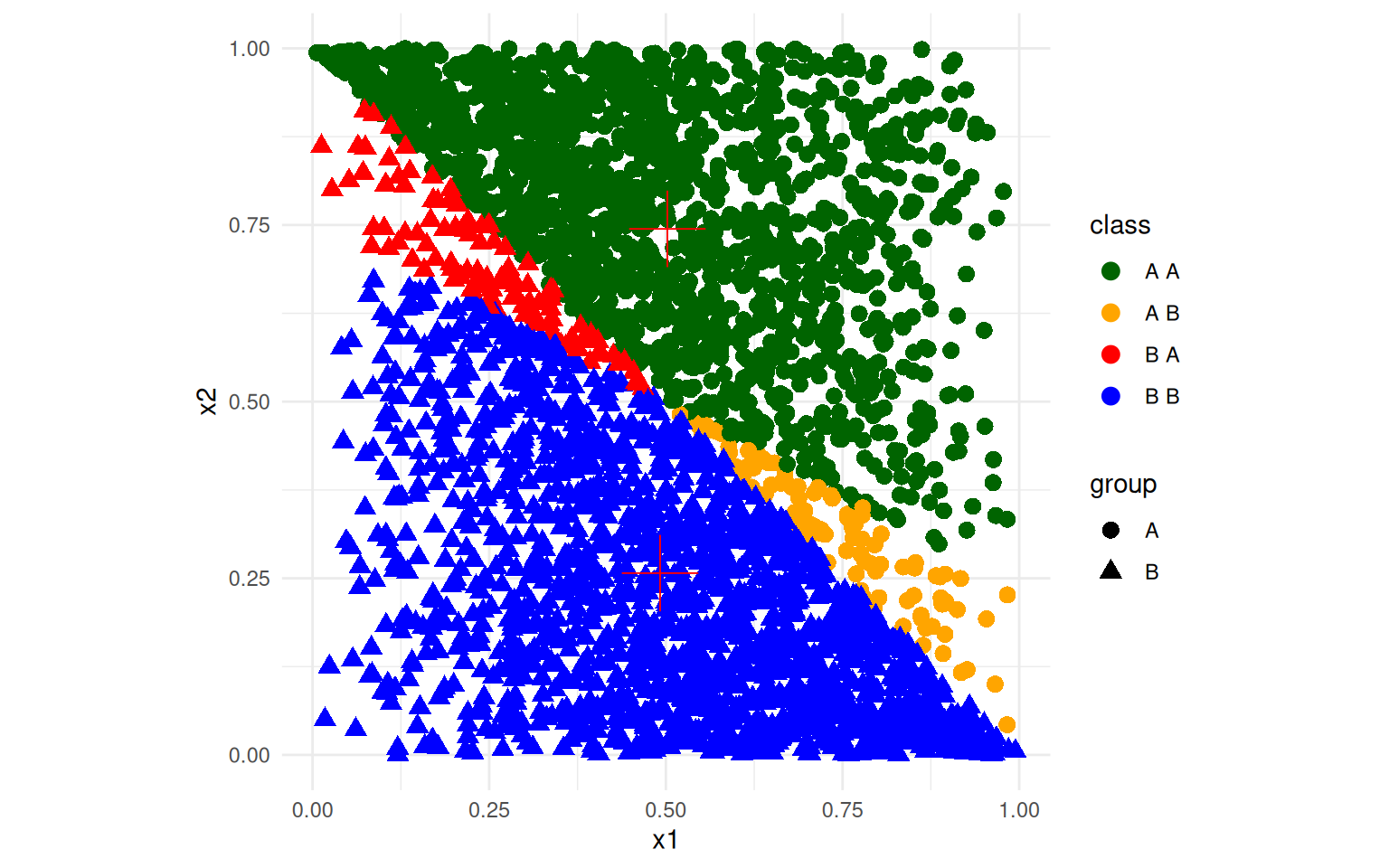In this grid search, no settings we looked at produced above-chance (above 50%) performance. This suggests that although the

## Exercise: iPhone data

phone <- read.csv("data_study1.csv")
phone$Smartphone <- factor(phone$Smartphone)
phone$Gender <- as.numeric(as.factor(phone$Gender))
phone.svm <- svm(y = phone$Smartphone, x = phone[, -1], scale = T, cross = 50) phone.svm  Call: svm.default(x = phone[, -1], y = phone$Smartphone, scale = T, cross = 50)

Parameters:
SVM-Type:  C-classification
cost:  1

Number of Support Vectors:  429
summary(phone.svm)

Call:
svm.default(x = phone[, -1], y = phone$Smartphone, scale = T, cross = 50) Parameters: SVM-Type: C-classification SVM-Kernel: radial cost: 1 Number of Support Vectors: 429 ( 227 202 ) Number of Classes: 2 Levels: Android iPhone 50-fold cross-validation on training data: Total Accuracy: 62.57089 Single Accuracies: 70 36.36364 70 63.63636 80 54.54545 63.63636 90 63.63636 50 54.54545 60 54.54545 100 60 72.72727 80 63.63636 72.72727 60 81.81818 80 54.54545 60 54.54545 36.36364 60 45.45455 80 72.72727 30 45.45455 63.63636 70 54.54545 70 72.72727 54.54545 70 45.45455 80 45.45455 60 81.81818 72.72727 50 54.54545 60 63.63636 45.45455  Here, we are about as good as other methods using cross-validation. It again would be worthwhile to select the C and gamma parameters via a grid search. ## Exercise: MNist data SVMs and the RBF kernel are especially well-suited for image data. We can use the image data, but this svm does not like features that have 0 variance, so let’s remove them. train <- (read.csv("trainmnist.csv")) var <- apply(train, 2, sd) train2 <- train[, var > 0] test <- as.matrix(read.csv("testmnist.csv")[, var > 0]) class <- as.factor(rep(c("0", "1"), each = 250)) mn.svm <- svm(y = class, x = train2, kernel = "radial", scale = F, cross = 10) table(class, predict(mn.svm, test))  class 0 1 0 249 1 1 2 248 # A trickier example This example comes from Andrew Ng’s open classroom tutorials on SVMs (http://openclassroom.stanford.edu/MainFolder/DocumentPage.php?course=MachineLearning&doc=exercises/ex8/ex8.html) ex4 <- read.csv("ex8a.csv", header = F) ex4$V1 <- as.factor(ex4$V1) ggplot(ex4, aes(x = V2, y = V3, colour = V1)) + geom_point(size = 3)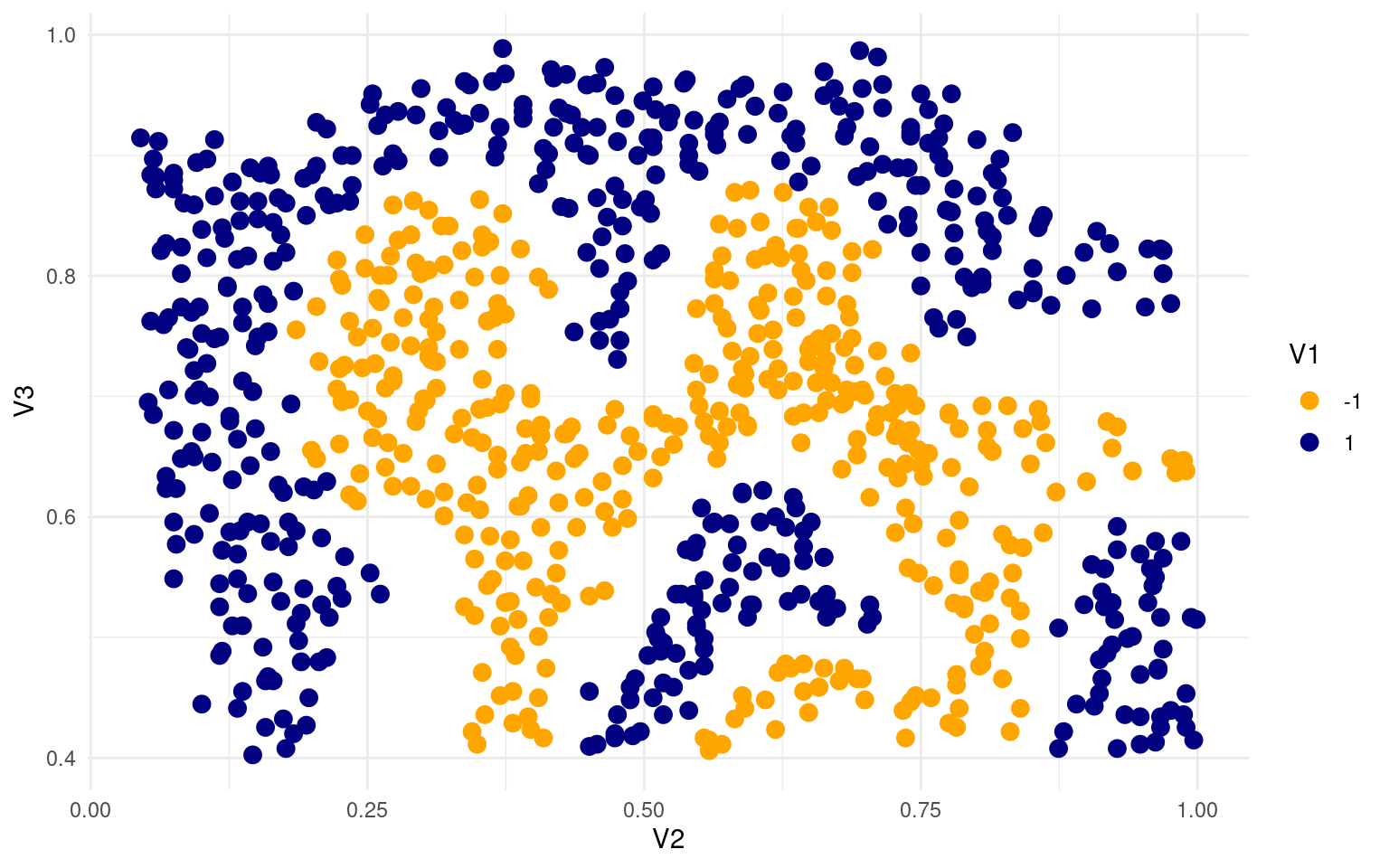Here, we can create a SVM that separates the two sets exactly. # set.seed(100) seed <- 2 set.seed(seed) svm.ng <- svm(V1 ~ ., data = ex4, kernel = "linear", scale = F, cross = 10, cost = 10, fitted = T) svm.ng  Call: svm(formula = V1 ~ ., data = ex4, kernel = "linear", cross = 10, cost = 10, fitted = T, scale = F) Parameters: SVM-Type: C-classification SVM-Kernel: linear cost: 10 Number of Support Vectors: 743 table(ex4$V1, predict(svm.ng))

-1   1
-1 177 206
1  166 314
ex4$class <- predict(svm.ng) ex4$class2 <- paste(ex4$V1, ex4$class)
ggplot(ex4, aes(x = V2, y = V3, colour = class2, shape = class)) + geom_point(size = 3)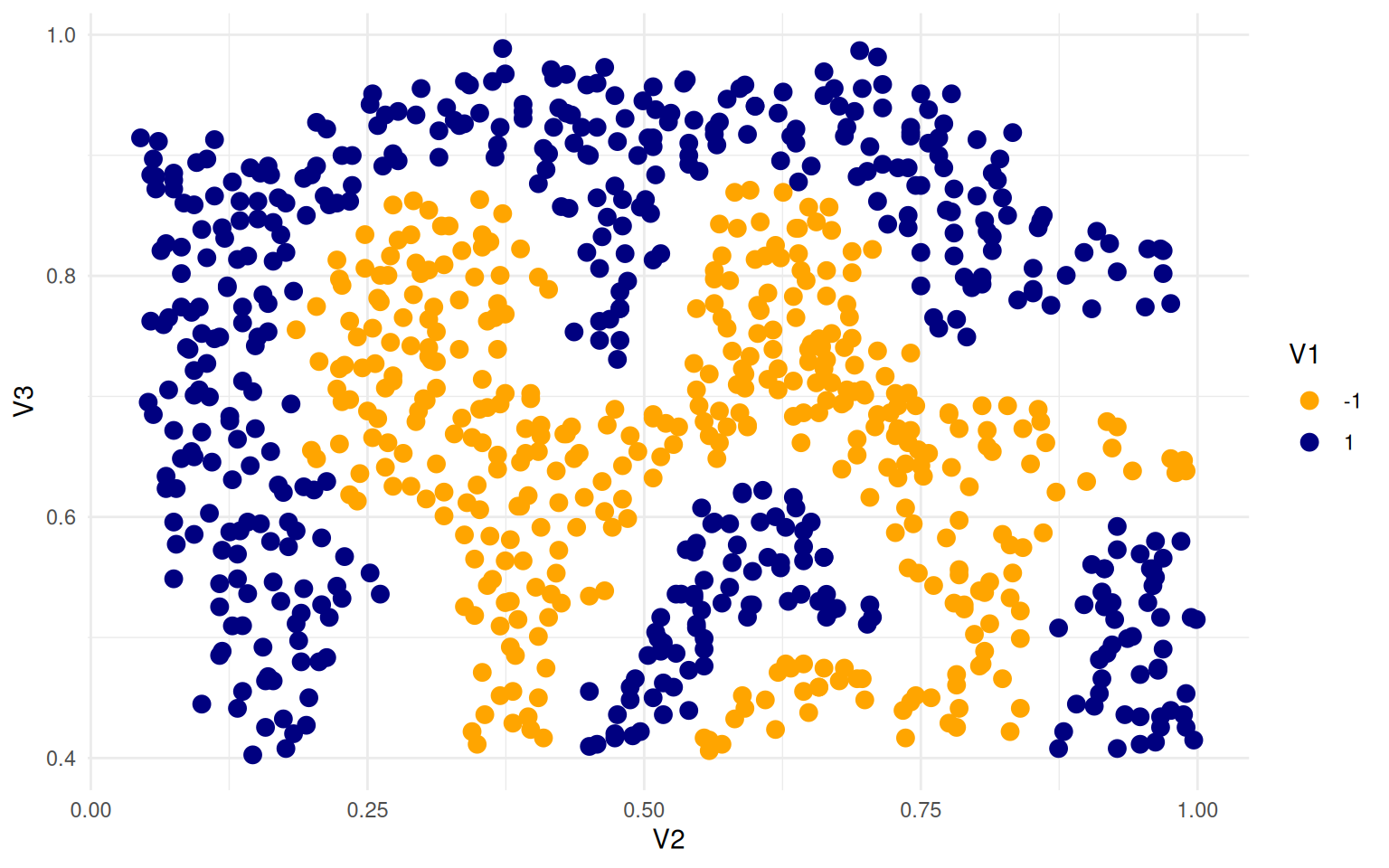This used a linear kernel. Every once in a while, this produces a bad solution, but generally it has perfect classification. If we try it again:

svm.ng <- svm(V1 ~ ., data = ex4, kernel = "linear", scale = F, cross = 10, cost = 10,
fitted = T)
svm.ng

Call:
svm(formula = V1 ~ ., data = ex4, kernel = "linear", cross = 10,
cost = 10, fitted = T, scale = F)

Parameters:
SVM-Type:  C-classification
SVM-Kernel:  linear
cost:  10

Number of Support Vectors:  12
table(ex4$V1, predict(svm.ng))  -1 1 -1 383 0 1 0 480 ex4$class <- predict(svm.ng)
ex4$class2 <- paste(ex4$V1, ex4$class) ggplot(ex4, aes(x = V2, y = V3, colour = class2, shape = class)) + geom_point(size = 3)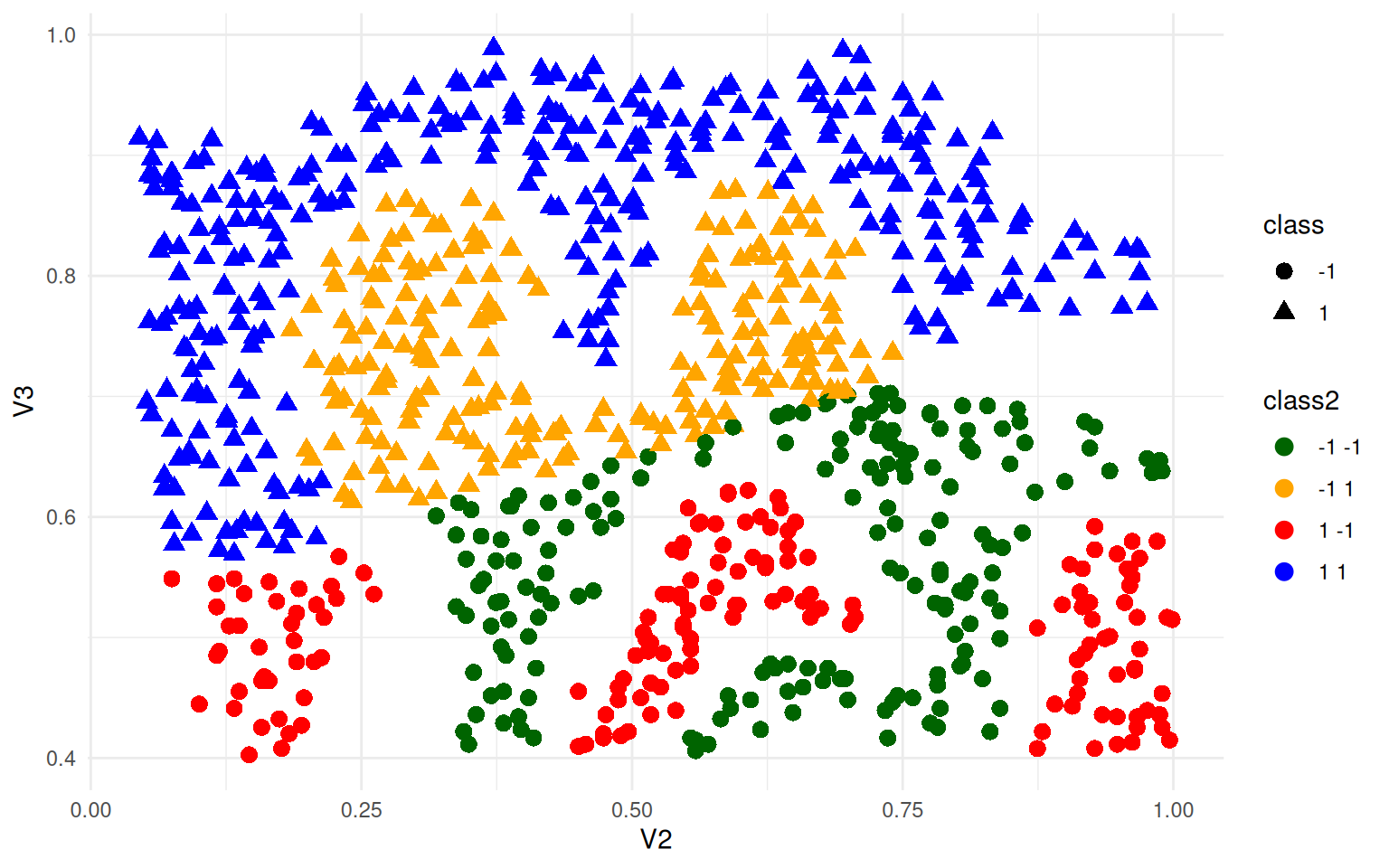What if we added some noise and re-trained? ex4b <- ex4 ex4b$V2 <- ex4b$V2 + rnorm(nrow(ex4), mean = 0, sd = 0.05) ex4b$V3 <- ex4b$V3 + rnorm(nrow(ex4), mean = 0, sd = 0.05) svm.ng <- svm(V1 ~ ., data = ex4b, kernel = "radial", scale = T, cross = 100, gamma = 100, cost = 0.5) svm.ng  Call: svm(formula = V1 ~ ., data = ex4b, kernel = "radial", cross = 100, gamma = 100, cost = 0.5, scale = T) Parameters: SVM-Type: C-classification SVM-Kernel: radial cost: 0.5 Number of Support Vectors: 804 table(ex4b$V1, predict(svm.ng))

-1   1
-1 383   0
1    0 480
ex4b$class <- predict(svm.ng) ex4b$class2 <- paste(ex4b$V1, ex4b$class)
ggplot(ex4b, aes(x = V2, y = V3, colour = class2, shape = class)) + geom_point(size = 3)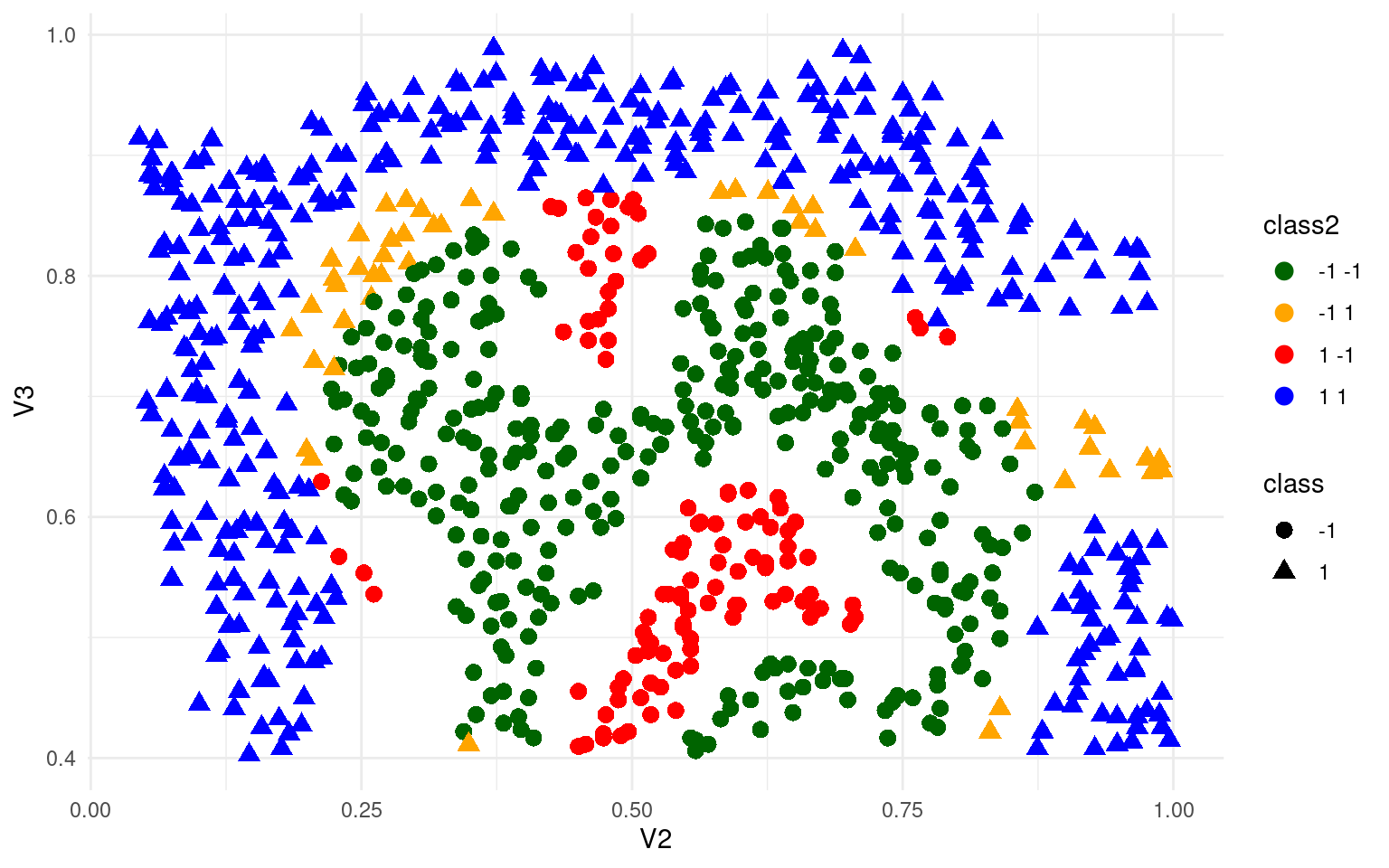We still get 100% accuracy still, even though we don’t have a clean overlap. This suggests that we have need to be careful to tune the parameters, as we clearly have over-fitting here but cannot really ameliorate it.